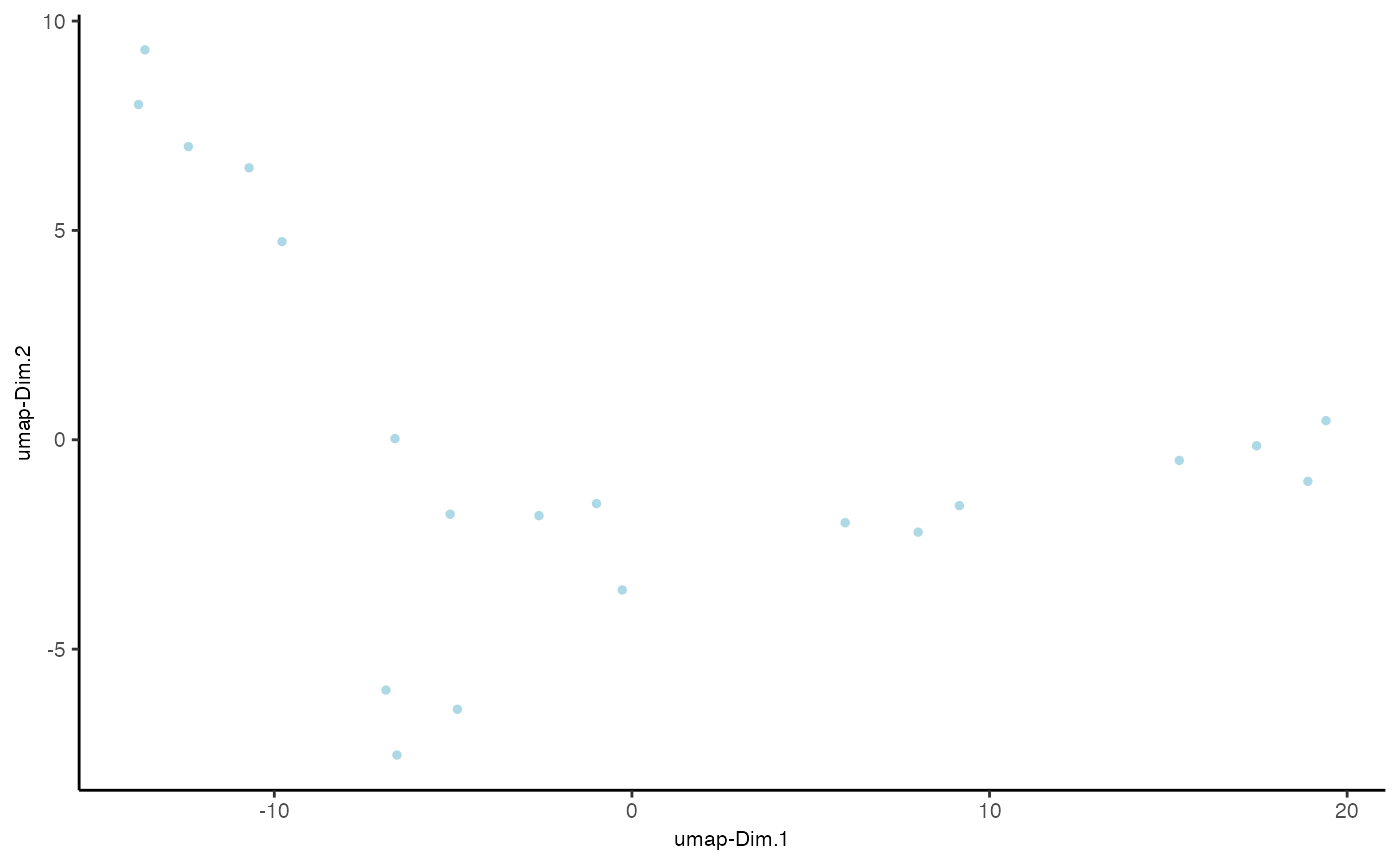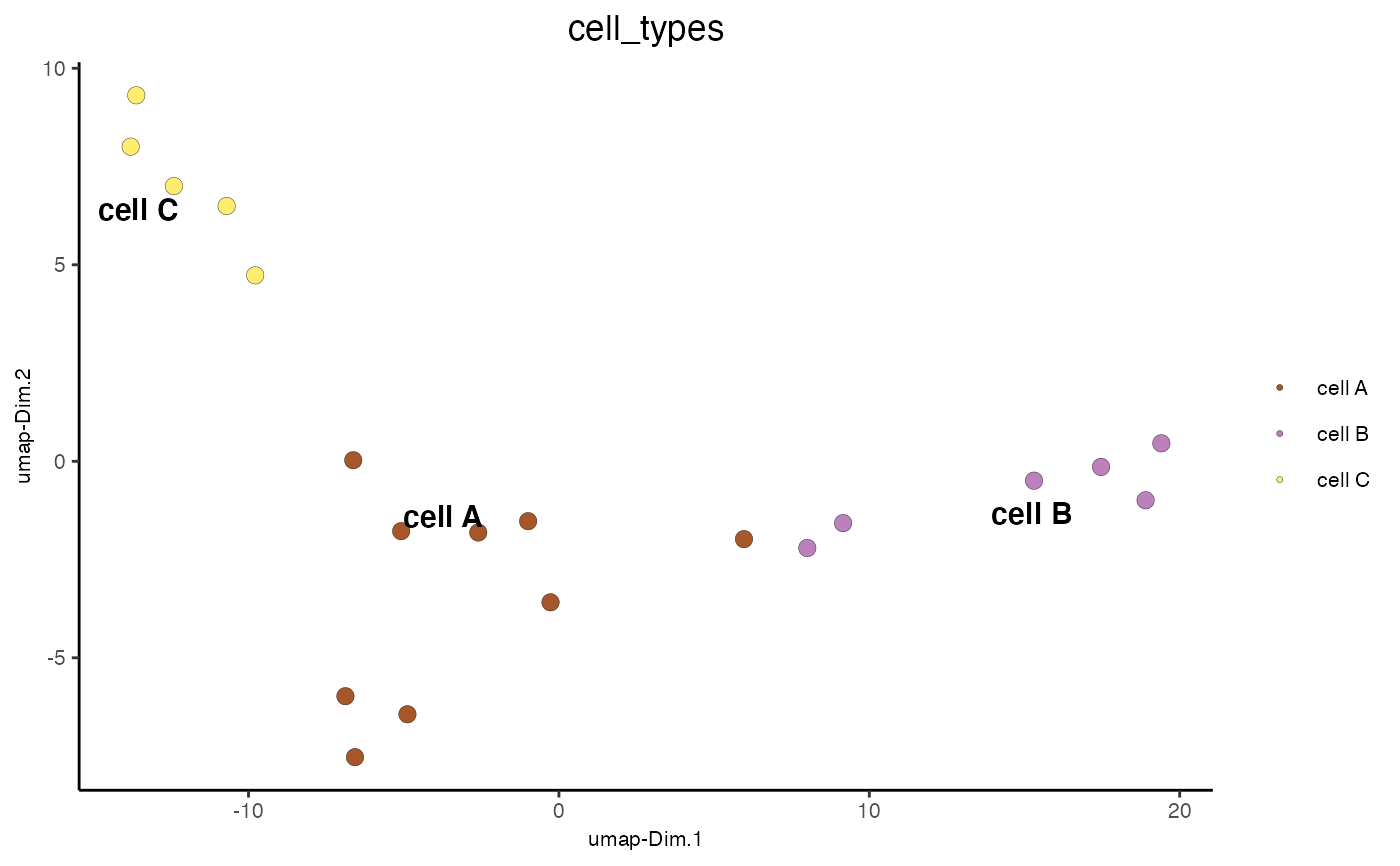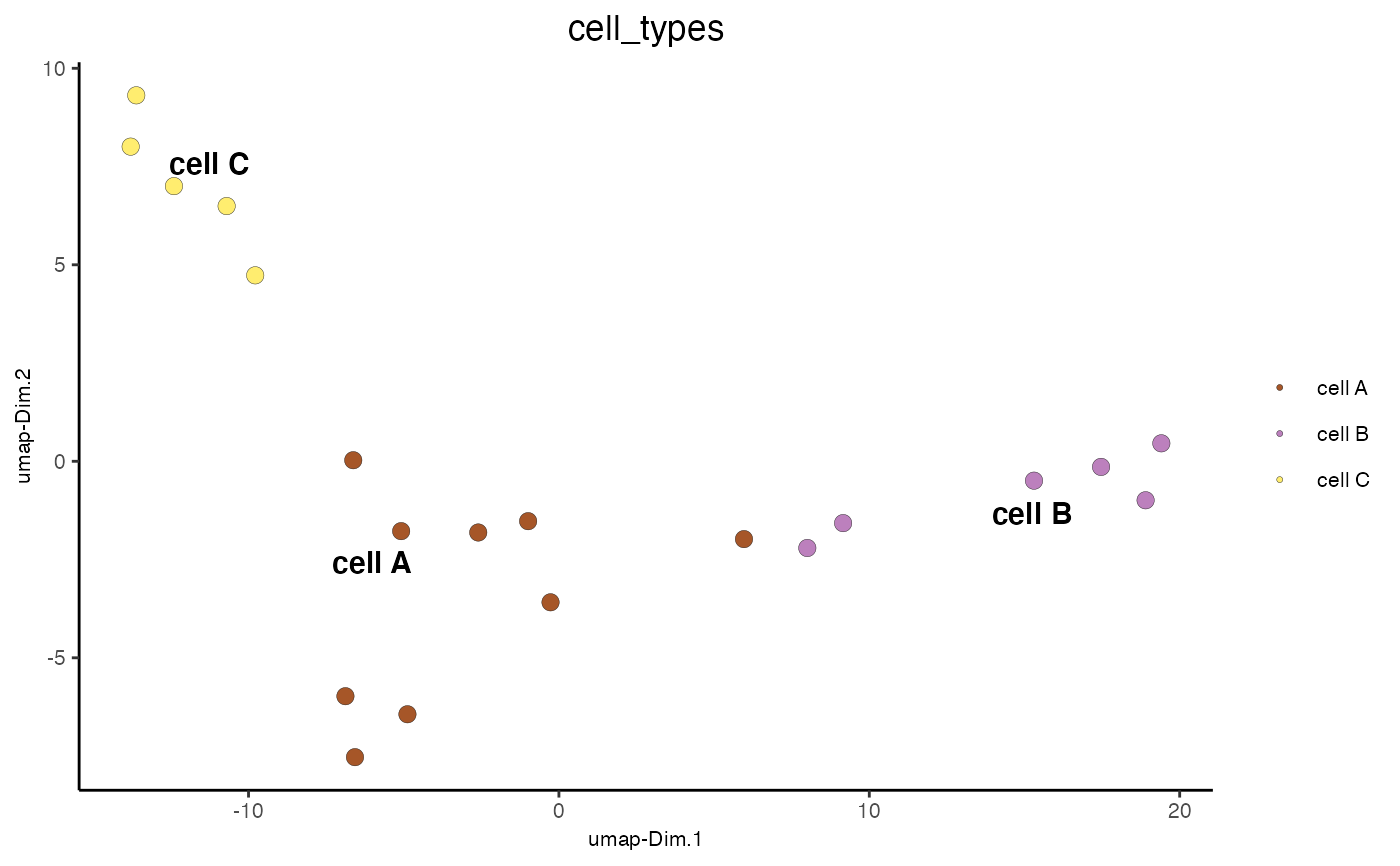Visualize cells according to dimension reduction coordinates

dimPlot2D(
gobject,
group_by = NULL,
group_by_subset = NULL,
dim_reduction_to_use = "umap",
dim_reduction_name = "umap",
dim1_to_use = 1,
dim2_to_use = 2,
spat_enr_names = NULL,
show_NN_network = F,
nn_network_to_use = "sNN",
network_name = "sNN.pca",
cell_color = NULL,
color_as_factor = T,
cell_color_code = NULL,
select_cell_groups = NULL,
select_cells = NULL,
show_other_cells = T,
other_cell_color = "lightgrey",
other_point_size = 0.5,
show_cluster_center = F,
show_center_label = T,
center_point_size = 4,
center_point_border_col = "black",
center_point_border_stroke = 0.1,
label_size = 4,
label_fontface = "bold",
edge_alpha = NULL,
point_shape = c("border", "no_border"),
point_size = 1,
point_alpha = 1,
point_border_col = "black",
point_border_stroke = 0.1,
title = NULL,
show_legend = T,
legend_text = 8,
legend_symbol_size = 1,
background_color = "white",
axis_text = 8,
axis_title = 8,
cow_n_col = 2,
cow_rel_h = 1,
cow_rel_w = 1,
cow_align = "h",
show_plot = NA,
return_plot = NA,
save_plot = NA,
save_param = list(),
default_save_name = "dimPlot2D"
)

## Arguments

gobject giotto object create multiple plots based on cell annotation column subset the group_by factor column dimension reduction to use dimension reduction name dimension to use on x-axis dimension to use on y-axis names of spatial enrichment results to include show underlying NN network type of NN network to use (kNN vs sNN) name of NN network to use, if show_NN_network = TRUE color for cells (see details) convert color column to factor named vector with colors vector with 3 colors for numeric data midpoint for color gradient vector with lower and upper limits select subset of cells/clusters based on cell_color parameter select subset of cells based on cell IDs display not selected cells color of not selected cells size of not selected cells plot center of selected clusters plot label of selected clusters size of center points border color of center points border stroke size of center points size of labels font of labels column to use for alpha of the edges point with border or not (border or no_border) size of point (cell) transparancy of point color of border around points stroke size of border around points title for plot, defaults to cell_color parameter show legend size of legend text size of legend symbols color of plot background size of axis text size of axis title cowplot param: how many columns cowplot param: relative height cowplot param: relative width cowplot param: how to align show plot return ggplot object directly save the plot [boolean] list of saving parameters, see showSaveParameters default save name for saving, don't change, change save_name in save_param

ggplot

## Details

Description of parameters. For 3D plots see dimPlot3D

Other reduced dimension visualizations: dimPlot3D(), dimPlot(), plotPCA_2D(), plotPCA_3D(), plotPCA(), plotTSNE_2D(), plotTSNE_3D(), plotTSNE(), plotUMAP_2D(), plotUMAP_3D(), plotUMAP()dimPlot2D(mini_giotto_single_cell, cell_color = 'cell_types', point_size = 3)